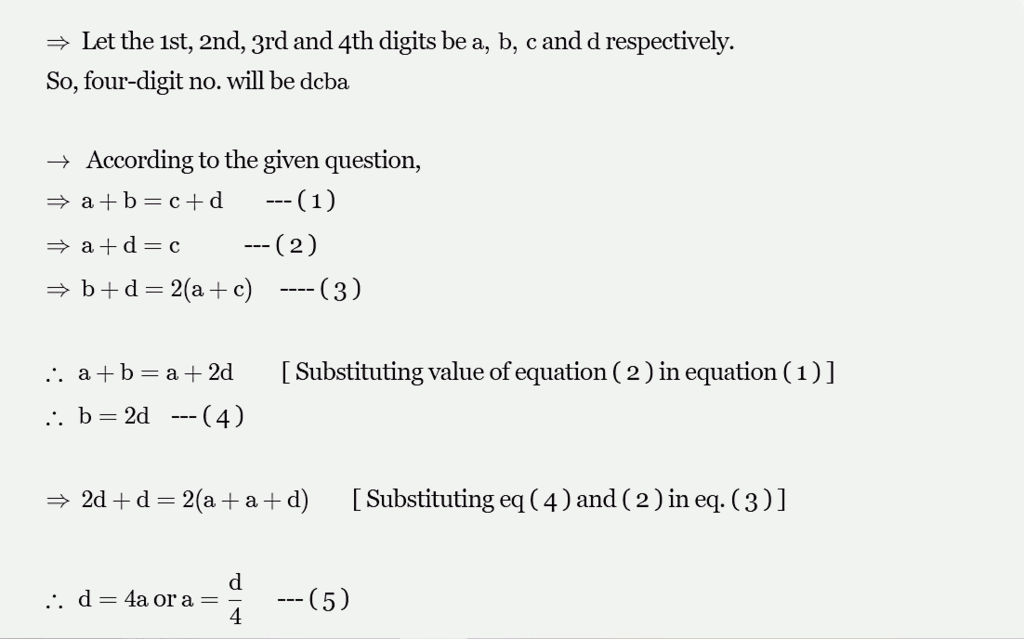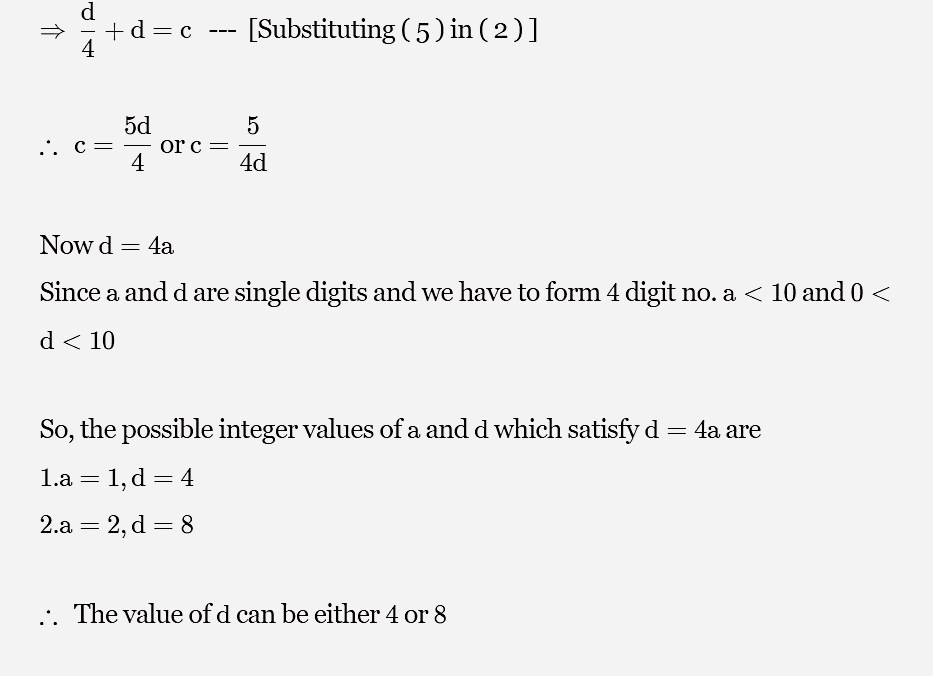In a four digit number the sum of first two digit is equal to that of the last two digits.The sum of the first and last digit is equal to the third digit . Finally the sum of the second and fourth digits is twice the sum of the other two digits what is the third digit of that number OPTIONS :- 1. FIVE 2.EIGHT 3.ONE 4. FOUR?Om Prakash Sep 06, 2021When

, then

.
When
, then

But the value of
should be less than
as it is a single digit.

Value of
would be
which is the third digit of the required number.

Answer :⇒ Whend=4, thenc=5.⇒ Whend=8, thenc=10.⇒ But the value ofcshould be less than10as it is asingle digit.∴ Value ofcwould be5which is the third digit of the required number.
Do you know? How Toppers prepare for CAT Exam
With help of the best CAT teachers & toppers, We have prepared a guide for student who are preparing for CAT : 15 Steps to clear CAT Exam